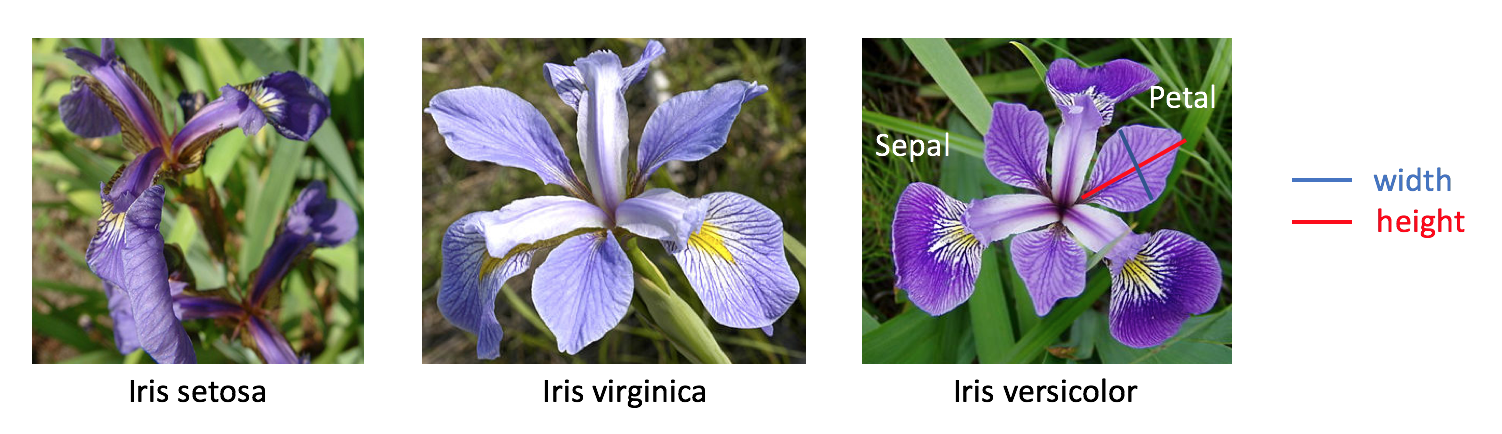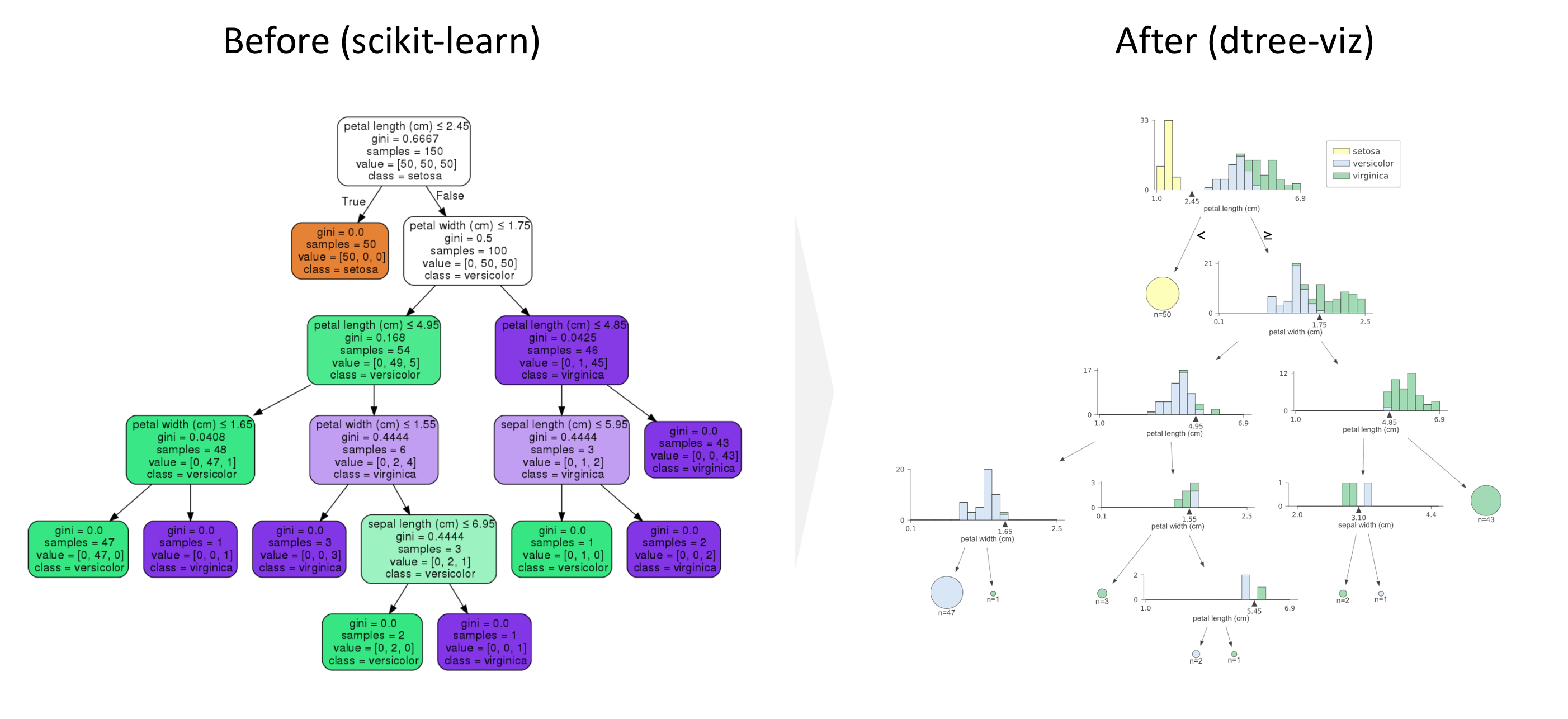# How to visualize a decision tree beyond scikit-learn

## Goal¶

The goal in this post is to introduce dtreeviz to visualize a decision tree for classification more nicely than what scikit-learn can visualize. We will walk through the tutorial for decision trees in Scikit-learn using iris data set.

Note that if we use a decision tree for regression, the visualization would be different.## Pre-requisite¶

First of install the module using pip or conda command as below.

In :
# !pip install dtreeviz
# ! pip install git+https://github.com/gautamkarnik/dtreeviz.git@update-for-cairo
# as of Apr, 5, 2019 need to use this pull request on Mac OSX 10.13. this pull request will be merged soon


In :
import pandas as pd
import numpy as np
from sklearn import tree

df_iris = pd.DataFrame(iris['data'],
columns=iris['feature_names'])
df_iris['target'] = iris['target']

Out:
sepal length (cm) sepal width (cm) petal length (cm) petal width (cm) target
0 5.1 3.5 1.4 0.2 0
1 4.9 3.0 1.4 0.2 0
2 4.7 3.2 1.3 0.2 0
3 4.6 3.1 1.5 0.2 0
4 5.0 3.6 1.4 0.2 0

## Train a Decision tree¶

In :
# Train the Decision tree model
clf = tree.DecisionTreeClassifier()
clf = clf.fit(iris.data, iris.target)


## Visualize a Decision Tree¶

### Scikit-learn¶

In :
import graphviz
dot_data = tree.export_graphviz(clf, out_file=None,
feature_names=iris.feature_names,
class_names=iris.target_names,
filled=True, rounded=True,
special_characters=True)

graph = graphviz.Source(dot_data)
graph

Out:

### dtreeviz¶

In :
from dtreeviz.trees import dtreeviz
viz = dtreeviz(clf,
iris['data'],
iris['target'],
target_name='',
feature_names=np.array(iris['feature_names']),
class_names={0:'setosa',1:'versicolor',2:'virginica'})

viz

Out:

What is better for drtreeviz

• You can see the distribution for each class at each node
• You can see where is the decision boundary for each split
• You can see the sample sie at each leaf as the size of the circle